# SSAT Upper Level Math : SSAT Upper Level Quantitative (Math)

## Example Questions

### Example Question #51 : Data Analysis / Probablility

A standard deck of 52 cards is altered by adding the jacks from another deck. What are the odds against a randomly drawn card being a club?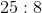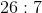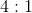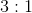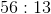Explanation:

Before the addition of the jacks, the deck had 13 clubs and 39 other cards. The 4 jacks from the other deck include 1 club and 3 other cards, so the altered deck contains 14 jacks and 42 other cards. That makes the odds against drawing a club at random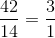- that is, 3 to 1, just as in the unaltered deck

### Example Question #51 : Data Analysis / Probablility

Which of the following ways can you alter a standard deck of 52 cards in order to neither increase nor decrease the probability that a randomly-drawn card would be a king?

Remove both of the black queens.

Remove the jacks.

Add the clubs from another deck.

Add the aces from another deck.

Add the clubs from another deck.

Explanation:

In an unaltered deck, 4 of the 52 cards, or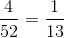of the cards, are kings. We are looking for an action that preserves this ratio.

We see that if the clubs from another deck are added, then there are 5 kings (the 4 original kings plus an added king) and 65 cards total (the original 53 plus the 13 added clubs). 5 of the 65 cards, or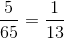of the cards, are kings, thereby preserving the ratio.

Of the other choices, none of them change the number of kings in the deck, but all change the total number of cards. Therefore, the ratio of kings to total cards must change.

### Example Question #1681 : Ssat Upper Level Quantitative (Math)

A standard deck of 53 cards (including the joker) is altered by adding the sevens from another deck. What are the odds against a randomly drawn card being a heart?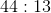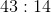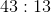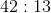Explanation:

Adding the four sevens from another deck to a 53-card deck will yield a 57-card deck with 14 hearts - the original 13 hearts and the added seven of hearts - and 43 other cards. Therefore, the odds against drawing a heart will be 43 to 14.

### Example Question #52 : Data Analysis / Probablility

Which of the following ways can you alter a standard deck of 52 cards in order to neither increase nor decrease the probability that a randomly-drawn card would be a spade?

None of the other responses gives a correct answer.

Remove the red fours and the black fives.

Add the red sevens from another deck.

Add the clubs from another deck.

Remove the red fours and the black fives.

Explanation:

In an unaltered deck, 13 of the 52 cards, or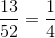of the cards, are spades. We are looking for an action that preserves this ratio.

If the red fours and the black fives, including the five of spades, are removed, then there are 12 spades left out of 48 cards, which means that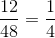of the cards are spades. This is the correct choice.

Of the other choices, none of them change the number of spades in the deck, but all change the total number of cards. Therefore, the ratio of spades to total cards must change.

### Example Question #81 : Data Analysis

Aaron, Gary, Craig, and Boone are sitting down in a row of four chairs. What is the probability that Aaron and Gary will be seated beside each other?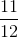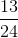Explanation:

Consider first all of the possible ways the men may be arranged, which is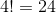Now, consider all of the ways that Aaron and Gary could be seated beside each other; it may be easier to visualize by drawing it out:

1. A G _ _
2. G A _ _
3. _ A G _
4. _ G A _
5. _ _ A G
6. _ _ G A

As seen, there are six possibilities.

Finally, for each of these cases, Craige and Boone could be seated in one of two ways.

So the probability that Aaron and Gary will be seated beside each other is: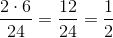### Example Question #52 : Data Analysis / Probablility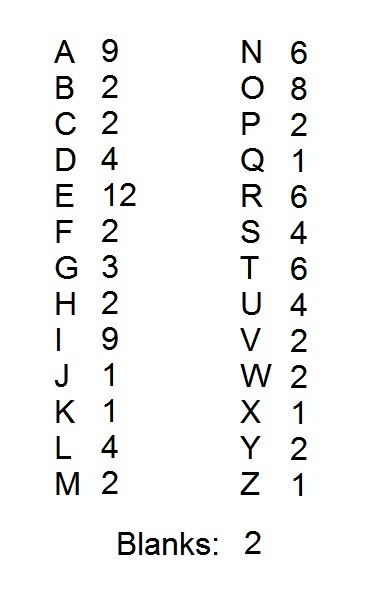A popular word game uses one hundred tiles, each of which is marked with a letter or a blank. The distribution of the tiles is shown above, with each letter paired with the number of tiles marked with that letter. Notice that there are two blank tiles.

All of the tiles are placed in a large box. What is the probability that a randomly drawn tile will be a vowel?

Note: for purposes of this question, "Y" is a consonant.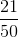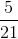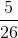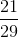Explanation:

Out of the 100 tiles, there are nine "A" tiles, twelve "E" tiles, nine "I" tiles, eight "O" tiles, and four "U" tiles. This is a total of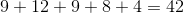tiles out of 100.

This makes the probability of drawing one of these tiles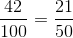### Example Question #51 : Data Analysis / Probablility

Eight standard decks of 52 cards are shuffled together; four jokers are shuffled in as well. A card is selected at random from this new deck. What is the probability that a spade will be selected?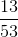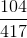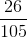Explanation:

Eight decks of 52 cards, with 4 jokers added, comprise a total of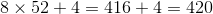cards. A standard deck of 52 cards has 13 spades, so the eight decks together have a total ofspades. This makes the probability of a random draw resulting in a spade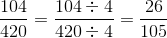### Example Question #51 : Data Analysis / Probablility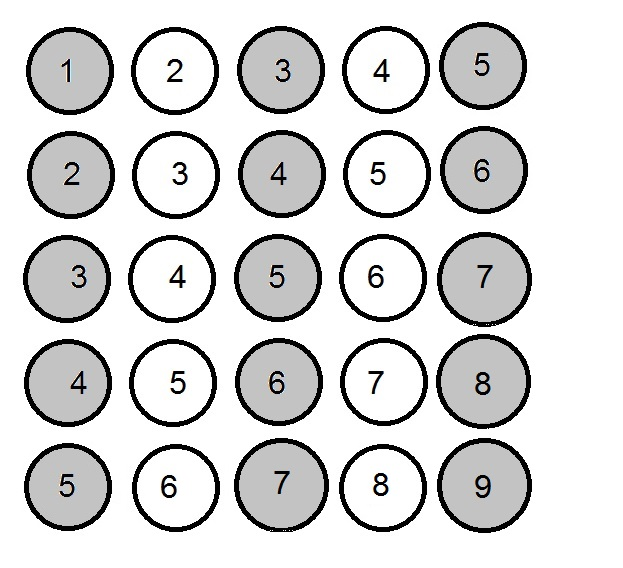The above set of balls is placed in a box. Give the probability that, if a ball is drawn at random, it will be a gray ball marked with an odd number.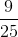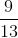Explanation:

As seen in the diagram below, nine of the twenty-five balls in the box are both gray and marked with odd numbers.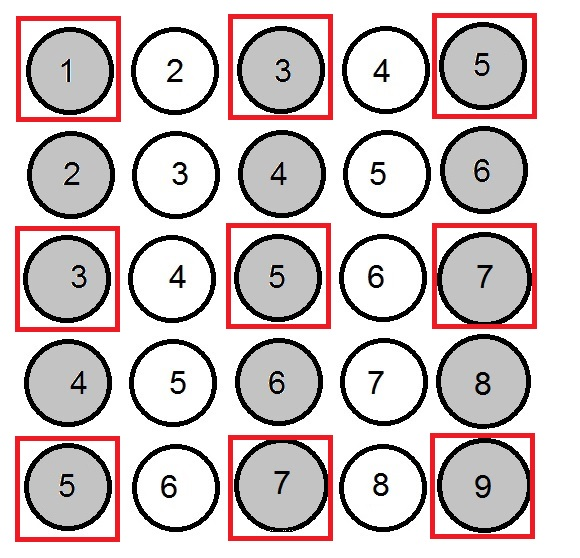This makes the probability that a random selection will yield one of these balls.

### Example Question #54 : Data Analysis / ProbablilityThe above set of balls is placed in a box. Give the probability that, if a ball is drawn at random, it will be a white ball marked with a prime number.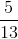Explanation:

Out of the whole numbers from 1 through 9, there are four prime numbers - 2, 3, 5, and 7. As seen in the diagram below, there are five out of twenty-five balls that are both white and marked with a prime number.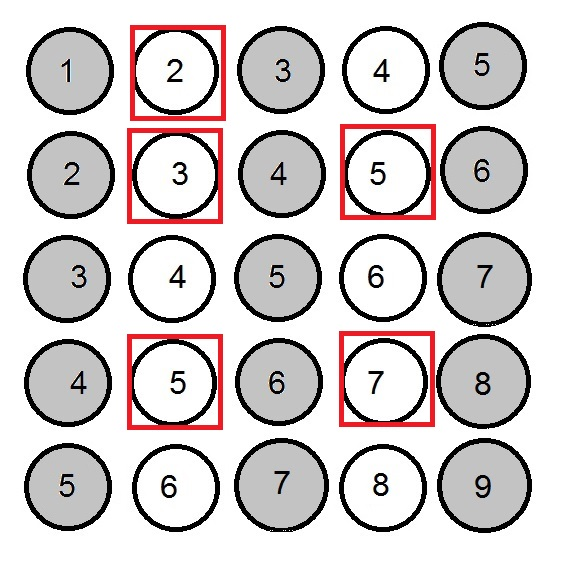This makes the probability of drawing one of these balls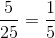.

### Example Question #55 : Data Analysis / Probablility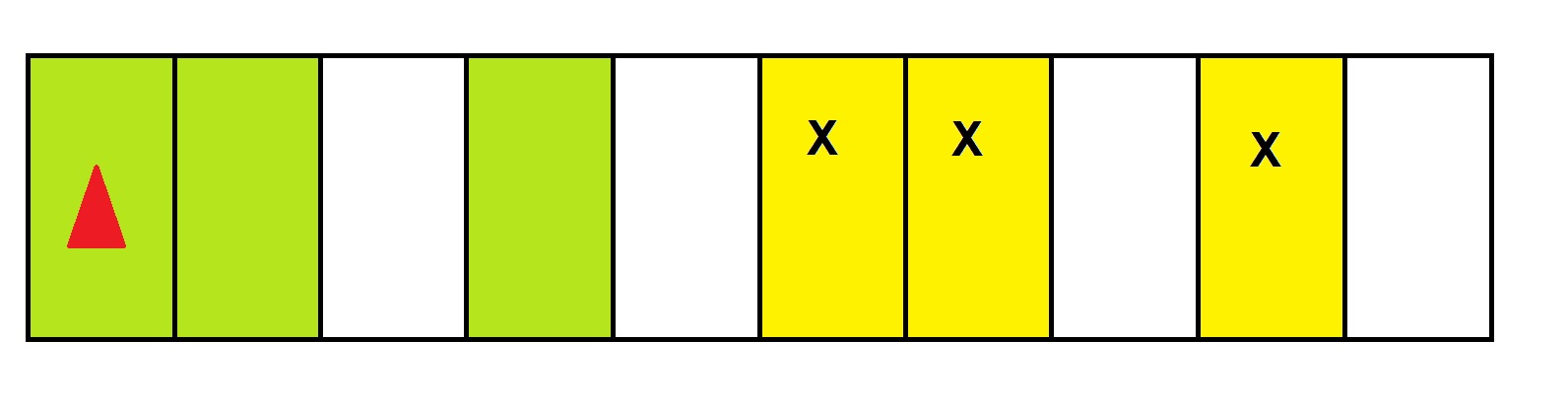Mark is playing a board game in which spaces represent property that can be "bought" and "sold"; the above diagram represents part of the board. Mark is represented by the red triangle, and he is moving in a left-to-right direction.

Mark is about to roll a pair of fair six-sided dice. If he lands on one of the yellow spaces, he will have to pay a huge fee to his opponent, Jennifer, who "owns" the properties represented by the spaces; if this happens, he will be out of the game.

What are the odds of this happening?

11 to 7 against

2 to 1 against

5 to 4 against

Even odds

11 to 7 against

Explanation:

The undesirable rolls are 5, 6, and 8. As can be seen in the diagram below, out of thirty-six equally probable outcomes possible with the roll of two fair six-sided dice, fourteen are undesirable, and twenty-two are desirable.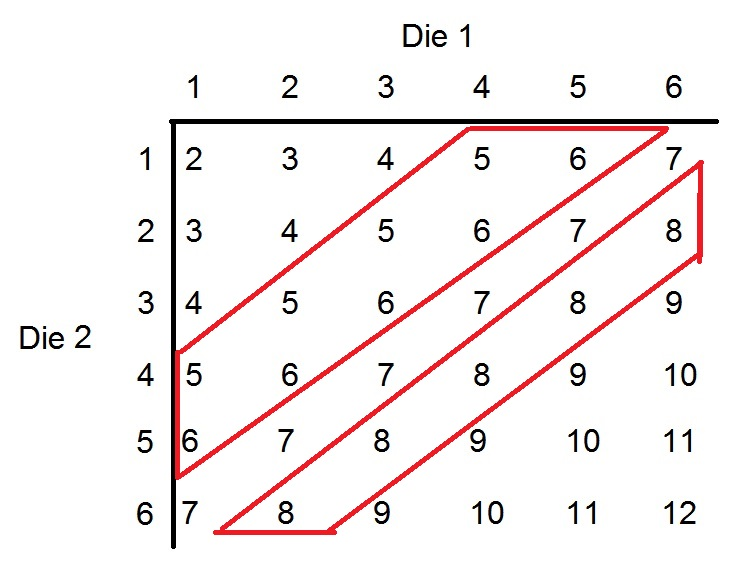The odds against an undesirable roll are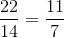- that is, 11 to 7.

### All SSAT Upper Level Math Resources